Email Us: info@lupinepublishers.comCall Us: +1 (914) 407-6109   57 West 57th Street, 3rd floor, New York - NY 10019, USA

# Lupine Publishers Group

## Lupine Publishers

Submit Manuscript

ISSN: 2641-6794# Genesis of the Planet Earth Volume 3 - Issue 5

Orlov S*

Petrozavodski State University, Petrozavodsk, Russia

Received: October 10, 2019;   Published: October 17, 2019

Corresponding author: Orlov S, Petrozavodski State University, Petrozavodsk, Russia

DOI: 10.32474/OAJESS.2019.03.000175

## Abstract

In this article we consider the origin and subsequent evolution of our planet. Theoretical studies are based on the author’s theory of vortex gravity, cosmology and cosmogony, and also on the law of conservation of the angular momentum of the body’s rotation. As a practical justification for calculations and conclusions, the well-known fact of slowing the rotation of the Earth is used. The principles of the formation of elementary particles and all types of inorganic and organic substances are proposed. The calculation of the physical state of our planet in the past and in the future is done. The age of the Earth is calculated in the article, which exceeds the generally accepted value by 1.2 billion years. It is shown that the principle of the origin and evolution of the Earth is fully applicable for the genesis of all other celestial bodies.

Keywords: Theory of vortex gravitation; Celestial mechanics; Cosmology; Cosmogony

## Introduction

From the 1600s and beyond people believed that the Earth’s rotation around its axis and revolves around the Sun has always happened and will continue. Doubts about the constancy of the speed of rotation of the earth arose after the discovery of E. Halley in 1695 observing secular acceleration of the moon’s motion. The idea of the secular slowing the rotation of the Earth under the influence of tidal friction was first proposed by Kant in 1755 Nowadays Richard Stephenson from Durham University in the UK, based on the descriptions of hundreds of solar and lunar eclipses for the last 2,700 years, came to the conclusion that the Earth continues to slow its rotation about its axis .

To cause slowing of the Earth are created by many factors. These include the gravitational influence of the moon and sun, braking or drag with the cosmic dust, atmospheric and geophysical processes and many other physical phenomena.

In this paper, conventional explanations of irregular rotation of the earth that is understood as an unproven and (or) is negligibly small. External gravitational influences or atmospheric effects can not only slow, but can also accelerate the Earth’s rotation. The genesis of our planet, including the slowing of its rotation, fully explained by the theory of vortex gravitation, cosmology and cosmogony  and the laws of mechanics. For greater clarity, the next chapter outlines the principles of the theory of vortex gravitation.

## The Theory of Vortex Gravitation

Vortex gravitation theory  is based on the well-known astronomical facts - most celestial objects revolve about an axis. The most logical explanation of the cause of this movement can be only one - the rotation of celestial objects spawned vortex rotation of cosmic matter – ether. Ether forms a global system of interconnected space vortices. Orbital velocity in each vortex ether (torsion), decreasing from the center to the periphery of the law of the inverse square of the removal. According to the laws of aerodynamics with Bernoulli’s’ Law - the slower the rate of fluid at a given pressure, the more pressure will occur. The pressure gradient generates a force pushing towards the zone with the lowest pressure - to the center of this torsion (Figure1). Thus, accumulates in the center of the torsion or created cosmic matter of which is generated by a celestial body. Consider the equation of vortex gravitation theory obtained in the theory of vortex gravitation, cosmology and cosmogony.

Figure 1: Two-dimensional model of the gravitational interaction between the two bodies.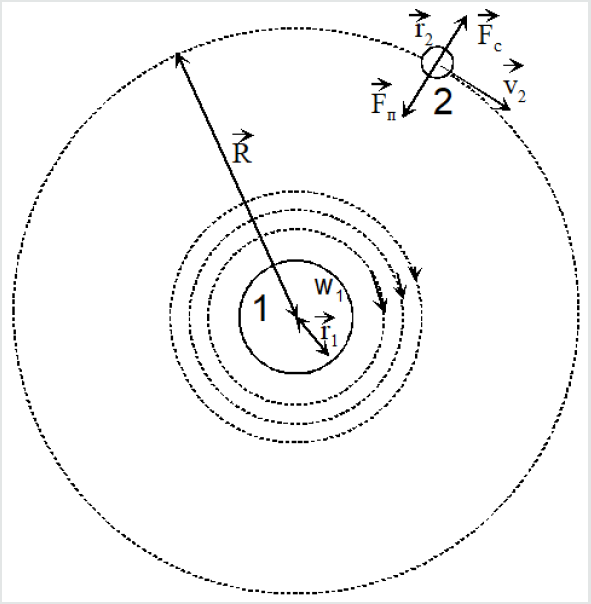Shown forces acting on the body 2. Fc - centrifugal force Fn - attraction force of body 2 to body 1, v2 - linear velocity of a body in orbit 2, R - radius of the orbit, r1 - radius of the body 1, r2 - radius of the body 2, w1 - the angular velocity of rotation of the ether on the surface of the body 1, m2 - mass of the body 2.

As already mentioned, the result of motion of the vortex pressure gradient arises. Radial distribution of pressure and velocity in the ether  defined on the basis of the Navier-Stokes equations for the motion of a viscous fluid (gas).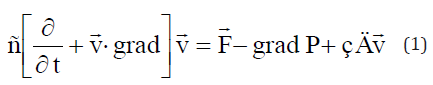in cylindrical coordinates, taking into account the radial symmetry vr = vz = 0 = v (r), P = P (r)j, equation can be written as a system.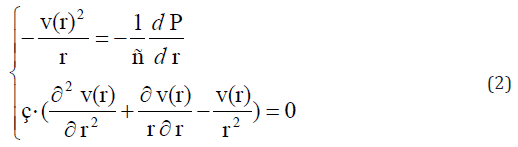where r = 8.85 x 10-12 kg / m3 - the density of , V - ether velocity vector, P - pressure ether, h - viscosity.

In cylindrical coordinates module gravitythen comparing (2) and (3) for an incompressible ether (r = const), we find that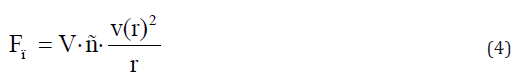• The assumption1: Ether pervades all space, including the physical body except Volume V in the formula (4) - an effective volume - the volume of the elementary particles that make up the body 2. All bodies are composed of electrons, protons and neutrons. Radius of the electron is much smaller than the radius of the proton and the neutron radius is approximately the same and the last of the order rn ~ 1.2 ∙ 10-15 m masses of the proton and neutron are approximately the same as mn ~ 1.67 ∙ 10-27 kg (rn, mn - the radius and mass of the nucleon).
• After the necessary transformations (full payment is set out in the theory vortex gravitation) is obtained:

A. Equation for determining the force of gravity depending on the rotational speed etherrn, mn - the radius and mass of the nucleon.

B. Equations for determining the dependence of the pressure on the body surface P0, the rotational speed ether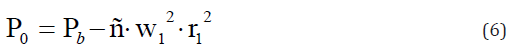where P0 - ether pressure at the surface of the body, using the boundary condition P( ∞) = Pb Pb-freeetherpressure.

Figure 2 is a graph showing the pressure distribution in accordance with formula (6).

## Changes in Volume and Mass of The Earth

According to the theory of vortex gravitation, cosmology and cosmogony, the initial moment of the appearance of any celestial body was the emergence of space, ether vortex. At the time of its inception, each vortex created his vortex gravity (Chapter 2). Vortex gravity can be regarded as a “generator world of matter” that sucks and (or) creates a vortex inside the elementary particles. Thus, the mass of each celestial object in the initial moment of its existence, was equal to the mass of the cosmic ether, from which it forms a vortex. Since the density of the ether is negligible, then the mass of each of the newly formed vortex ether should be close to zero. Consequently, each celestial body in the history of its existence, increased its weight from “zero conditional” to these values. It is worth noting an important condition - cosmic whirlwind unopposed, constantly maintained and retains its original speed (see assumption. № 1). Therefore, the space whirlwind constantly generates the same mass of substance. That is any celestial body increases the weight by a constant.

Modern studies customary slowing the Earth’s rotation value 0.00002 seconds in each year . Then the relative increase in time for the Earth’s rotation around its axis is increased by a factor Kt.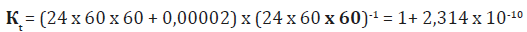By increasing the time for one revolution of the earth that is inversely proportional to the rotational speed decreases Ve. Then the relative decrease in the rate of rotation of Kv can be expressed asFurther calculations are based on the law of conservation of angular momentum the Earth’s rotation around its axis.

M Vv Re = const (8)

M-massoftheplanet,
Vv-thespeedofrotationoftheplanet,
From equation (8)

M Vv Re = (Km M) (Kvv Vv) (Kre Re) = const

Where the coefficients Km, Kvv, Kre - show the relative changes in the values M, Vv, Re.
Hence

Km Kvv Kre = 1 (9)

Substituting (7) into (9) we obtain

Km Kre = 1 + 2,314 х 10-10 (10)

Planet mass (M), as well as its volume (V) is proportional to the radius of the planet in the cube.

M ~ V ~ Re3 here

Kre3 = Km (11)

Substituting (11) into (10)

Kr4 = 1+ 2,314 х 10-10 or

Kr = (1 + 2,314 х 10-10)1/4 = 1 + 5,785 х 10-11 (12)

Substituting (12) into (10) we determine the relative increase in the mass of the Earth –

Km = 1+ 1,735 х 10-10 (13)

### To Determine the Absolute Values of the Physical Characteristics of the Earth

• At rotation speed of the Earth surface at the equator Vv = 465,1 m/s annual slowdown will

465,1 х 2,314 х 10-10 = 9,3 х 10-8 м/sek (14)

• When the radius of the Earth Re = 6371000 m annual increase in the radius of the Earth

6371000 х 5,785 х 10-11 = 3,7 х 10- 4 м или 0,37 мм/year (15)

or 37cm in 1,000 years or 370m 1 000 000 years

Therefore, the earth deposits at a depth of about 370 m have an average age of the sediments million years ago.

• When the mass of the Earth M = 9736 × 1024 kg annual weight gain

5,9736 х 1024 х 1.735 х 10-10 = 1,036 х 1015 kg (16)

• Increased Earth

The surface area of the Earth Sv = 4 M R2. Increasing the radius of - 3.7 x 10-4m
Then the volume of the Earth increases by –

4 П R2 х 3,7 х 10-4 = 4 х 3.14 х (6,371 х 106)2 х 3,7 х 10-4 = 1,886 х 1011 м3 (17)

P = 3,456 х 1014 х (1,835 х 1011)-1= 1883 kg/м3 (18)

Note. The absolute values of the above characteristics of the Earth is only valid in the present historical moment, as the speed, radius and mass of the planet are constantly changing.

## Mass and Age of The Earth, Orbital and Radial Motion

Earth's massis constantly increasingthe amount of(13)

Km = 1.735 x 10-10 x M

No outside forces act on the planet. Therefore, legitimate to use the law of conservation of angular momentum of the Earth around the Sun.

M Vo Ro = const, where

Km K vo K ro = 1 (19)

M - mass of the Earth

Vo - the orbital speed of the Earth

Ro - radius of Earth's orbit.

Km, Kvo, Kro - rate of change in the Earth's mass units, the speed of its revolution around the Sun and the orbit radius.

According to Kepler's law V ~ Ro-1/2, we can write

Kvo = Kr-1/2 (20)

Substitute (20) into (19)

Kro = Km-2 = (1 + 1.735 х 10-10)-2 = (1 + 3,47 х 10-10)-1 (21)

Substituting (21) and (13) into equation (19) we obtain

Kvo = (Km x Kro)-1 = (1 + 3,47 х 10-10) x (1+1.735 х 10-10)-1 = 1 + 1.735 х 10-10 (22)

Mass of the planet increases always constant. Therefore, by dividing the mass of the Earth on its permanent annual increase (M x 1.735 x 10-10), we obtain the age of the planet

Т = M х (М х Km)-1 = M х (M x 1.735 х 10-10)-1 = (1,735 х 10-10)-1 = 5,76 billion years

Radioisotope dating  established the age of the planet size of 4.54 billion years. It should be noted that the radioisotope dating explored only the surface layers of the planet. Therefore, the results of these studies (age) can be attributed only to the same surface layers.

The proposed method of determining the age of the planet considering as a single physical object, which increases the reliability of its results.

Annual approaching the Earth's orbit to the Sun

Ro x Kro = 15 х 1010 x 3,47 х 10-10 = 52,05 m/year

One revolution of the Earth around the sun (one year) is reduced by:

365,24 (day) х 24 х 60 х 60 х 1,735 х 10-10 = 0,0054 sec/year

## Past and The Future of The Planet

Calculation of physical properties in the past and the future.

### 5.1 1 000 000 000 years ago

The Rotation of the Planet:

Mass of the planet billion years ago - 1,036 x 1015 (kg) x (4.76 x 109) (s) = 4.93 x 1024kg

Mass ratio - Km-1 = 4.93 x 1024/5, 97 x 1024 = 0,826

The relative change Earth radius - Kr-1 = (Km-1) 1/3 = (o, 826)1/3 = 0.94

From equation (19), - Kv-1 = (Kr x Km-1) -1 = (0.826 x 0.94) -1 = 1.288

Absolute values of the planet a billion years ago. Since the velocity of the planet was more than 1,288 times in,

Length of day a billion years ago - 18.6 hours. Radius of the planet was less than 0.94 times or

R-1 = 6,371 х 106 х 0,94 = 5,988 х 106 м.

Consequently, the force of gravity was greater in (0.94) -1/2 = (0.88)-1 times. That is – F-1 = 11,1m

Handling the planet

Using formulas 20, 21, 22 and Km-1 = 0.826, we find:

1. The ratio ofthe radii ofK r0-1 = 466
2. The relative decrease inorbital velocity(1billion years ago) – Kvо-1 = 0,826
3. The orbital speed V0-1 = 29,8 х 103 х 0,826 = 24,6 х 103 m/s

Ro-1= R x Kro-1= 15 x 1010 x 1,466 = 219,9mln km

The number of days (18.6 hours). Per revolution (one year) - 838,4 days.

### After 1 000 000 000 Years in the Future

The rotation of the planet:

Mass of the planet a billion years - 1,036 х 1015 (kg) х 6,76 х 109(years) = 7,0 х 1024 kg

Changing the masses - Km+1 = 7,0 х 1024/5,97 х 1024 = 1,173

The relative change radius of the Earth - Kr+1= (Km+1)1/3= (1,173)1/3 = 1,055

From equation (19), - Kv+1= (Kr+1 x Km+1)-1= (1,055 х 1,173)-1 = 0,81

The absolute values of the properties of the planet in the future in a billion years. Since the rotation speed of the planets will be less than 0.81 times, then

Length of the day - 29.6 hours

Radius of the planet - R +1 = 6,721 x 106m

The gravitational force F = 8,8m

Handling the Planet:

Using formulas 20, 21, 22 and Km +1 = 1,173, we find:

1. The relative increase inorbital velocity(inbillion yearsin the future) Kvо+1 = 1,042
2. relation of orbital radiuses Kro+1 = 0,727

Ro+1= R x Kro+1= 15 x 1010 x 0,727= 109mln km

1. The orbital speed of V0 + 1 = 29.8 x 103 x 1,042 x 103 = 31.05m / s
2. Number of days (29.6 hours) per revolution (year) - 206.9

Note 1: The proposed calculation of the radius of Earth's orbit are valid only if the orbital plane is considered a celestial body (the Earth) coincides with the center plane of the gravitational aether vortex. In the event of the orbital plane of the celestial body of the plane gravitational torsion vortex gravitation force is reduced in proportion to the cube of the cosine of the deviation. A detailed calculation of this dependence is presented in the theory of vortex gravitation.

Orbital plane of the Earth's gravity deviates from the plane by 1 degree. Consequently, the tops of the small semiaxes earth orbit force swirl solar gravity decreases by 0.045% in comparison with the magnitude of gravity, which was determined by the classical equations. Therefore, changing the radius of the orbit of the Earth or the duration of the year in the past or the future, calculated in this article actually have smaller value.

For large deviations of the orbital planes of any celestial object and the long duration of their existence can remove the orbital trajectories of these objects from the call center. Such removal (38 mm per year) is currently seen in the moon.

## Creation of Substance of The Planet

Most modern scholars explain the increased mass of the planet and meteorite dust flux of cosmic matter on Earth. The magnitude of this cosmic matter is determined by researchers in the order of several tens of thousands of tons (107 kg) per year. In this paper we calculated that the observed slowing rotation of the planet can only be achieved by increasing the mass of the planet at 1,036 x 1015kg per year (the equation 16). This calculated weight exceeds the estimated mass of cosmic matter that falls to Earth from space, hundreds of millions of times. Consequently, the total mass of cosmic dust and meteorites falling annually on our planet, is negligible Therefore, the study of increasing the mass of the Earth, the mass of meteorites can be neglected and consider another source of creation of matter.

Based on the principles of vortex gravitation and cosmogony - the substance of all celestial bodies (elementary particles) create ethereal vortices.

Consider the principles of vortex cosmogony. According to the theory of ether-dynamics , the following parameters of the ether:

1. Pressure in the quiet, motionless air – 2x1032nm-2
2. Ether density r = 8.85x10-12kg/m3

On the basis of equation (6) at a speed of ether v0 = 4,75 x 1021 Earth torsion pressure should drop to zero (Figure2). We define the radius of the orbit of the Earth torsion with zero pressure.

On the basis of equation (5) determine the rotational velocity of the ether on the Earth's surface is ve = 1.277 x 1018 m/c. Transform equation (20) -

V20 / v2e = re / r0, where

ve = 1.277х1018м/c - The orbital velocity of the ether on the surface of the Earth

v0 = 4,75х1021м/c – ether velocity in orbit with zero pressure

re = 6371000m - radius of the Earth

r0 - earth orbit radius of the vortex, where the pressure is zero (no orbit).
Substituting the known values of v0, ve, re, define r0.

r0 = 0,46m

Zero pressure in the Earth's orbit to any torsion means cessation of ether motion on this orbit, which caused reduction (increase) in ether pressure to the initial value in the quiescent state - 2 x 1032 N m-2.

Note. Meteorologists note full tranquility (calm) in the center of tropical or sea storms. These phenomena are called "a storm eye".

The sharp increase in pressure in the central orbits torsion in these areas will create a vortex anti gravity force directed from the center of the torsion of the orbit with zero pressure (46cm).

Thus, on the above, the zero orbit the Earth torsion, on both sides, there are two huge forces over the vortex gravitation. From equation (5) determine the gravitational force (F0) in the Earth's orbit on the torsion bar with a radius of 0.46m.

F0 = 1,9 x 1015 m

Two opposite forces of gravity give rise to corresponding compression forces that cause the seal over rarefied ether to super dense state. Thus, in the center of any cosmic torsion created over dense core, which does not pass through it no radiation, including air. In the downtown core - the emptiness. At the same time, the core material is impermeable barrier to the orbital rotation of the ether on the orbits of the orbit below the outer surface of the core. When driving close to the surface of the ether in its core flow turbulence arises, twist and many micro vortices. These micro vortices similar planetary torsion, its own gravity pulls and seals the outer gaseous. In the center of micro-torsions particle density reaches the nucleon density - 1017 kg/m3. It should be noted that the establishment of micro-torsions occur at higher orbits the Earth torsion than the orbit of the nucleus. Vortex gravitation force decreases with increasing orbit. This allows not only nucleons, but also atoms. Continuously generated by the atoms of various substances in the central space of the vortex, generate celestial bodies. Throughout the history of the mega all the heavenly bodies the formation of atoms - continuous. Pouring into the magma, the newly formed atoms constantly "feed" the heavenly bodies more weight. Magma periodically overflowing through the Earth's crust cracks comes to the surface as lava. Geologists estimate the total mass of lava poured out a year tens of cubic kilometers, or 1012 cubic meters. The average population density in the order of lava 103kg/m3, its mass is measured about 1015kg.

Consequently, the actual mass of lava poured out annually, representing an increase of mass of the planet, according to the proposed settlement. This proves the accuracy of the proposed study on age and change in the physical parameters of the Earth. The mere appearance of the substance (lava) from the depths of the planet proves that the substance appeared in the bowels of the earth and was not delivered from space. This statement is obvious as meteorites or a space dust can't get in a planet body. Continuous creation of substance from ether in a planet possibly only at continuous inflow of ether to the planetary torsion. We will determine the volume of ether necessary for creation of 1015 kg of planetary substance.

Increases in mass of Earth has to be equal to the mass of ether from which this additional mass of a planet was created. If we share quantity of annual increase in mass of Earth into ether density, we will determine the volume of ether which annually is required for substance weighing 1015 creation.

Ve = m/r = 1015 / 8.85 x 10-12 = 1,13 x 1026 m3

This volume corresponds to the volume of the ether’s torsion with a radius of 1 million km and with an axial thickness of 100 thousand km.

All of the above principles of vortex cosmogony apply to all celestial bodies in our universe. The main conclusion of Chapter 6 - the substance is created by cosmic vortices of ether inside of celestial bodies. Moving the matter in the universe from one celestial body to another has only a minor character and the physical properties of celestial bodies affect.

Generated in the Earth's core atoms of chemical elements are constantly overwhelmed this kernel and create a giant pressure overlying layers of the planet. This pressure exceeds in magnitude the opposite direction to him the gravitational pull of the Earth. Thus, the overlying layers of the earth continuously shifted by the lower layers towards the surface. During this movement, the chemical elements at certain depths enter the geophysical processes and form we all known inorganic compound - rock, hydrocarbons, water, and other gases. At a certain moment in history, these deposits are close to the earth's crust, or come to the surface of the Earth. Consequently, all the necessary human civilization minerals - inexhaustible. In place of the explored and develop hydrocarbons, diamonds, gold and other precious natural resources for humans, the Earth will create new and thrust them out of their depth in the crust or on the surface.

The proposed principles of inorganic matter, just as likely to apply to the creation of an organic substance. Only certain earth formations are extreme chemical and physical conditions which permit the synthesis of organic molecules and their compounds. Obviously, on the surface of planets create conditions impossible. Consequently, there cannot be a synthesis of organic matter.

Organic matter, since its inception in the depths of the heavenly bodies, in certain historical moments erupts on the surface of the Earth, together with inorganic substances. For the development of the organic substance to a variety of animal and plant species on the Earth's surface requires the existence of favorable natural conditions, primarily these include optimal vortex gravitation force on the surface of the planet and the solar heat and light exposure.

Not only plant and animal species emerged from the bowels of the planet, but also pathogenic viruses and bacteria, as evidenced by the annals of weather disasters and epidemics. Message from Thucydides (c. 460-400 BC) is known, that the epidemic that raged in Attica between 436 and 427 years. BC, accompanied by strong earthquakes, sea floods, droughts and crop failures. In Europe, after the eruption of Etna in 1333, broke out "Black Death" - the bubonic and pneumonic plague, bevel most of the population of medieval Europe (about 60 million). In article A Chizhevsky  noted numerous cases of the relationship of animal diseases and plant protection and catastrophic atmospheric phenomena.

Consequently, most commonly known to mankind world catastrophes (floods, glaciation, pandemics, tectonic movements of the crust, etc.), as well as the emergence and transformation of the atmosphere, hydrosphere, biosphere, topography and climate occurred and are occurring as a result of emissions to the surface of terrestrial matter. Meteorites and cosmic dust have on the development of the biosphere is only minor and insignificant impact.

The basis of all organic and inorganic matter - ether. For the development of air into living or non-living body, that is, for their origin and evolution are necessary extreme conditions that can only be created inside a celestial body and the corresponding vortex. Thus, space, ether vortices - creators of all known forms and types of matter.

1. (2008) Journal for the History of Astronomy. pp. 229.
2. S Orlov (2012) Foundation of vortex gravitation, cosmology and cosmogony. Global journal of science Frontier research. Physic and Space Science 12(1): 1-21.
3. VA Atsurovskiy (1990) General ether-dynamics. Energo atomizdat. Russia, p. 278.
4. Dalrymple G Brent (2001) The age of the Earth in the twentieth century: A problem (mostly)solved. Special Publications, Geological Society of London 190(1): 205-221.
5. AL Chizhevsky (1930) Epidemic catastrophes and periodic activity of the Sun. p. 172.
Close## Online Submission System

### Submit ManuscriptClick here for Manuscript Sample Templates### Drag and drop files here

or

( For multiple files submission, zip them in a single file to submit. For file zipping software Download )